International
Tables for
Crystallography
Volume B
Reciprocal space
Edited by U. Shmueli

International Tables for Crystallography (2006). Vol. B, ch. 5.1, p. 546   | 1 | 2 |

## Section 5.1.7.1.2. Absorbing crystals

A. Authiera*

aLaboratoire de Minéralogie-Cristallographie, Université P. et M. Curie, 4 Place Jussieu, F-75252 Paris CEDEX 05, France
Correspondence e-mail: authier@lmcp.jussieu.fr

#### 5.1.7.1.2. Absorbing crystals

| top | pdf |

Rocking curve. Since the sign of γ is negative,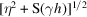in (5.1.3.10)has a very large imaginary part when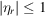. It cannot be calculated using the same approximations as in the Laue case. Let us set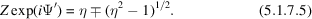The reflecting power is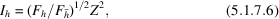where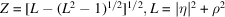and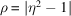is the modulus of expression (5.1.7.5)where the sign is chosen in such a way that Z is smaller than 1.

The expression for the reflected intensity in the absorbing Bragg case was first given by Prins (1930). The way of representing it given here was first used by Hirsch & Ramachandran (1950). The properties of the rocking curve have been described by Fingerland (1971).

There is no longer a total-reflection domain and energy penetrates the crystal at all incidence angles, although with a very high absorption coefficient within the domain. Fig. 5.1.7.2gives an example of a rocking curve for a thick absorbing crystal. It was first observed by Renninger (1955). The shape is asymmetric and is due to the anomalous-absorption effect: it is lower than normal on the low-angle side, which is associated with wavefields belonging to branch 1 of the dispersion surface, and larger than normal on the high-angle side, which is associated with branch 2 wavefields. The amount of asymmetry depends on the value of the ratio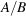of the coefficients in the expression for the imaginary part of the deviation parameter in (5.1.3.7): the smaller this ratio, the more important the asymmetry.Figure 5.1.7.2 | top | pdf |Theoretical rocking curve in the reflection case for a thick absorbing crystal. The 400 reflection of GaAs using Cu Kα radiation is shown.

Absorption coefficient. The effective absorption coefficient, taking into account both the Borrmann effect and extinction, is given by (Authier, 1986)where β is defined in equation (5.1.3.7)and Ψ′ in equation (5.1.7.5), and where the sign is chosen in such a way that Z converges. Fig. 5.1.7.3shows the variation of the penetration depth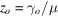with the deviation parameter.Figure 5.1.7.3 | top | pdf |Bragg case: thick crystals. Variation of the penetration depth with incidence angle (represented here by the dimensionless deviation parameter η). Thin curve: without absorption; thick curve: with absorption for the 400 reflection of GaAs using Cu Kα radiation.

### References

Authier, A. (1986). Angular dependence of the absorption induced nodal plane shifts of X-ray stationary waves. Acta Cryst. A42, 414–426.Google Scholar
Fingerland, A. (1971). Some properties of the single crystal rocking curve in the Bragg case. Acta Cryst. A27, 280–284.Google Scholar
Hirsch, P. B. & Ramachandran, G. N. (1950). Intensity of X-ray reflection from perfect and mosaic absorbing crystals. Acta Cryst. 3, 187–194.Google Scholar
Prins, J. A. (1930). Die Reflexion von Röntgenstrahlen an absorbierenden idealen Kristallen. Z. Phys. 63, 477–493.Google Scholar
Renninger, M. (1955). Messungen zur Röntgenstrahl-Optik des Idealkristalls. I. Bestätigung der Darwin–Ewald–Prins–Kohler-Kurve. Acta Cryst. 8, 597–606.Google Scholar